《The rsync algorithm》这篇发表于 1996 年的论文中介绍了一种名为 rsync 的增量同步算法，它能够快速地将两个文件夹中的内容同步。该算法利用了文件的局部性和差异性，通过计算文件的弱校验和和块校验和来确定文件的相似性，并进行增量同步。该算法具有高效性、可靠性和安全性等优点，在实际应用中被广泛使用。

## 0、摘要

This report presents an algorithm for updating a file on one machine to be identical to a file on another machine. We assume that the two machines are connected by a low-bandwidth high-latency bi-directional communications link. The algorithm identifies parts of the source file which are identical to some part of the destination file, and only sends those parts which cannot be matched in this way. Effectively, the algorithm computes a set of differences without having both files on the same machine. The algorithm works best when the files are similar, but will also function correctly and reasonably efficiently when the files are quite different.

## 1、问题

Imagine you have two files, A and B, and you wish to update B to be the same as A. The obvious method is to copy A onto B.

Now imagine that the two files are on machines connected by a slow com- munications link, for example a dial up IP link. If A is large, copying A onto B will be slow. To make it faster you could compress A before sending it, but that will usually only gain a factor of 2 to 4.

Now assume that A and B are quite similar, perhaps both derived from the same original file. To really speed things up you would need to take advantage of this similarity. A common method is to send just the differences between A and B down the link and then use this list of differences to reconstruct the file.

The problem is that the normal methods for creating a set of differences between two files rely on being able to read both files. Thus they require that both files are available beforehand at one end of the link. If they are not both available on the same machine, these algorithms cannot be used (once you had copied the file over, you wouldn’t need the differences). This is the problem that rsync addresses.

The rsync algorithm efficiently computes which parts of a source file match some part of an existing destination file. These parts need not be sent across the link; all that is needed is a reference to the part of the destination file. Only parts of the source file which are not matched in this way need to be sent verbatim. The receiver can then construct a copy of the source file using the references to parts of the existing destination file and the verbatim material.

rsync 算法能够高效地计算源文件与目标文件中匹配的部分。 这部分数据不需要通过链接发送；所需要的只是引用目标文件的部分数据。 只需要发送源文件中不匹配的分布数据。然后，接收者可以使用对现有目标文件部分内容的引用和逐字记录的材料（传输的差异数据）来构建源文件的副本。

Trivially, the data sent to the receiver can be compressed using any of a range of common compression algorithms, for further speed improvements.

## 2、rsync算法

Suppose we have two general purpose computers a and b. Computer a has access to a file A and b has access to file B, where A and B are “similar”. There is a slow communications link between a and b.

The rsync algorithm consists of the following steps:

rsync算法包括以下步骤：

1. b splits the file B into a series of non-overlapping fixed-sized blocks of size S bytes  . The last block may be shorter than S bytes.
2. For each of these blocks b calculates two checksums: a weak “rolling” 32-bit checksum (described below) and a strong 128-bit MD4 checksum.
3. b sends these checksums to a.
4. a searches through A to find all blocks of length S bytes (at any offset, not just multiples of S) that have the same weak and strong checksum as one of the blocks of B. This can be done in a single pass very quickly using a special property of the rolling checksum described below.
5. a sends b a sequence of instructions for constructing a copy of A. Each instruction is either a reference to a block of B, or literal data. Literal data is sent only for those sections of A which did not match any of the blocks of B.

1. b 将文件 B 拆分为一系列大小为 S 字节  的非重叠的固定大小的块。 最后一个块的大小可能小于 S 字节。
2. 对于这些块中的每一个，b 会计算两个校验和：弱 “滚动” 32 位校验和（如下所述）和强 128 位 MD4 校验和。
3. b 将这些校验和发送给 a。
4. a 搜索 A 以找到所有长度为 S 字节的块（在任何偏移量，而不仅仅是 S 的倍数），这些块具有与 B 的块之一相同的弱校验和和强校验和。使用下面介绍的滚动校验和的特殊属性可以非常快速地一次完成此操作。
5. a 向 b 发送一系列指令，用于构造 A 的副本。每条指令要么是对 B 块的引用，要么是文字数据。 只有当 A 和 B 的不匹配的部分数据块才会发送文字数据。

The end result is that b gets a copy of A, but only the pieces of A that are not found in B (plus a small amount of data for checksums and block indexes) are sent over the link. The algorithm also only requires one round trip, which minimises the impact of the link latency.

The most important details of the algorithm are the rolling checksum and the associated multi-alternate search mechanism which allows the all-offsets checksum search to proceed very quickly. These will be discussed in greater detail below.

## 3、滚动校验和

The weak rolling checksum used in the rsync algorithm needs to have the property that it is very cheap to calculate the checksum of a buffer X(2)..X(n+1) given the checksum of buffer X(1)..X(n) and the values of the bytes X(1) and X(n+1).

rsync 算法中使用的弱滚动校验和必须具有以下特性：在给定缓冲区 X(1) .. X(n) 的校验和的情况下，计算缓冲区 X(2) .. X(n +1) 的校验和以及字节 X(1) 和 X(n + 1) 非常方便 。

The weak checksum algorithm we used in our implementation was inspired by Mark Adler’s adler-32 checksum. Our checksum is defined by: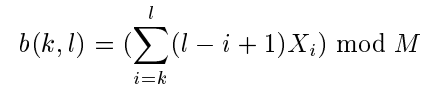where s(k, l) is the rolling checksum of the bytes X(k)..X(l). For simplicity and speed, we use M = 2^16 .

The important property of this checksum is that successive values can be computed very efficiently using the recurrence relation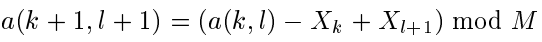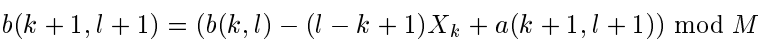Thus the checksum can be calculated for blocks of length S at all possible offsets within a file in a “rolling” fashion, with very little computation at each point.

Despite its simplicity, this checksum was found to be quite adequate as a rst level check for a match of two file blocks. We have found in practice that the probability of this checksum matching when the blocks are not equal is quite low. This is important because the much more expensive strong checksum must be calculated for each block where the weak checksum matches.

## 4、校验和搜索

Once a has received the list of checksums of the blocks of B, it must search A for any blocks at any offset that match the checksum of some block of B. The basic strategy is to compute the 32-bit rolling checksum for a block of length S starting at each byte of A in turn, and for each checksum, search the list for a match. To do this our implementation uses a simple 3 level searching scheme.

The first level uses a 16-bit hash of the 32-bit rolling checksum and a 2^16 entry hash table. The list of checksum values (i.e., the checksums from the blocks of B) is sorted according to the 16-bit hash of the 32-bit rolling checksum. Each entry in the hash table points to the first element of the list for that hash value, or contains a null value if no element of the list has that hash value.

At each offset in the file the 32-bit rolling checksum and its 16-bit hash are calculated. If the hash table entry for that hash value is not a null value, the second level check is invoked.

The third level check involves calculating the strong checksum for the current offset in the file and comparing it with the strong checksum value in the current list entry. If the two strong checksums match, we assume that we have found a block of A which matches a block of B. In fact the blocks could be different, but the probability of this is microscopic, and in practice this is a reasonable assumption.

When a match is found, a sends b the data in A between the current file offset and the end of the previous match, followed by the index of the block in B that matched. This data is sent immediately a match is found, which allows us to overlap the communication with further computation.

If no match is found at a given offset in the file, the rolling checksum is updated to the next offset and the search proceeds. If a match is found, the search is restarted at the end of the matched block. This strategy saves a considerable amount of computation for the common case where the two files are nearly identical. In addition, it would be a simple matter to encode the block indexes as runs, for the common case where a portion of A matches a series of blocks of B in order.

## 5、流水线

The above sections describe the process for constructing a copy of one file on a remote system. If we have a several files to copy, we can gain a considerable latency advantage by pipelining the process.

This involves b initiating two independent processes. One of the processes generates and sends the checksums to a while the other receives the difference information from a and reconstructs the files.

If the communications link is buffered then these two processes can proceed independently and the link should be kept fully utilised in both directions for most of the time.

## 6、结果

To test the algorithm, tar files were created of the Linux kernel sources for two versions of the kernel. The two kernel versions were 1.99.10 and 2.0.0. These tar files are approximately 24MB in size and are separated by 5 released patch levels.

Out of the 2441 files in the 1.99.10 release, 291 files had changed in the 2.0.0 release, 19 files had been removed and 25 files had been added.

A “diff” of the two tar files using the standard GNU diff utility produced over 32 thousand lines of output totalling 2.1 MB.

The following table shows the results for rsync between the two files with a varying block size.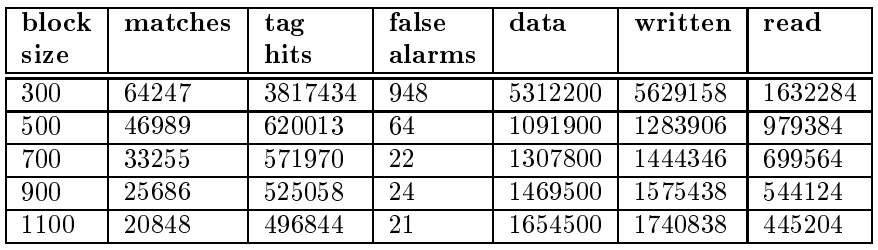In each case, the CPU time taken was less than the time it takes to run “diff” on the two files. 

The columns in the table are as follows:

• block size : The size in bytes of the checksummed blocks.
• matches : The number of times a block of B was found in A.
• tag hits : The number of times the 16 bit hash of the rolling checksum matched a hash of one of the checksums from B.
• false alarms : The number of times the 32 bit rolling checksum matched but the strong checksum didn’t.
• data : The amount of file data transferred verbatim, in bytes.
• written : The total number of bytes written by a including protocol overheads. This is almost all file data.
• read : The total number of bytes read by a including protocol overheads. This is almost all checksum information.

• 块大小 ： 校验和块的大小（以字节为单位）。
• 匹配数 ： 在 A 中找到 B 块的次数。
• 标签点击数 ： 滚动校验和的 16 位散列与来自 B 的校验和之一的散列匹配的次数。
• 误报 ：32 位滚动校验和匹配但强校验和不匹配的次数。
• 数据 ： 逐字传输的文件数据量，以字节为单位。
• 写 ： 包括协议开销在内的写入的总字节数。 这几乎是所有文件数据。
• 读 ： 包括协议开销在内的读取的总字节数。 这几乎就是所有的校验和信息。

The results demonstrate that for block sizes above 300 bytes, only a small fraction (around 5%) of the file was transferred. The amount transferred was also considerably less than the size of the diff file that would have been transferred if the diff/patch method of updating a remote file was used.

The checksums themselves took up a considerable amount of space, although much less than the size of the data transferred in each case. Each pair of checksums consumes 20 bytes: 4 bytes for the rolling checksum plus 16 bytes for the 128-bit MD4 checksum.

The number of false alarms was less than 1=1000 of the number of true matches, indicating that the 32 bit rolling checksum is quite good at screening out false matches.

The number of tag hits indicates that the second level of the checksum search algorithm was invoked about once every 50 characters. This is quite high because the total number of blocks in the file is a large fraction of the size of the tag hash table. For smaller files we would expect the tag hit rate to be much closer to the number of matches. For extremely large files, we should probably increase the size of the hash table.

The next table shows similar results for a much smaller set of files. In this case the files were not packed into a tar file first. Rather, rsync was invoked with an option to recursively descend the directory tree. The files used were from two source releases of another software package called Samba. The total source code size is 1.7 MB and the diff between the two releases is 4155 lines long totalling 120 kB.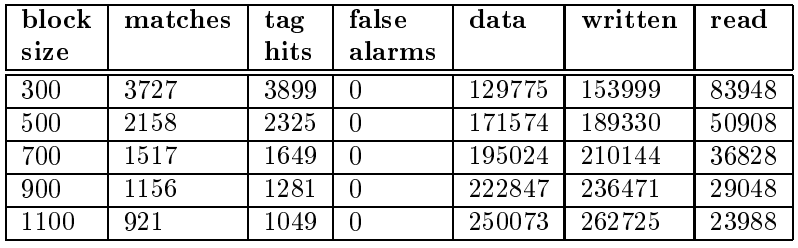## 7、可用性

An implementation of rsync which provides a convenient interface similar to the common UNIX command rcp has been written and is available for download from ftp://samba.anu.edu.au/pub/rsync.

rsync 的实现提供了一个类似于通用 UNIX 命令 rcp 的方便接口，已经编写完成，可以从 ftp://samba.anu.edu.au/pub/rsync 下载。